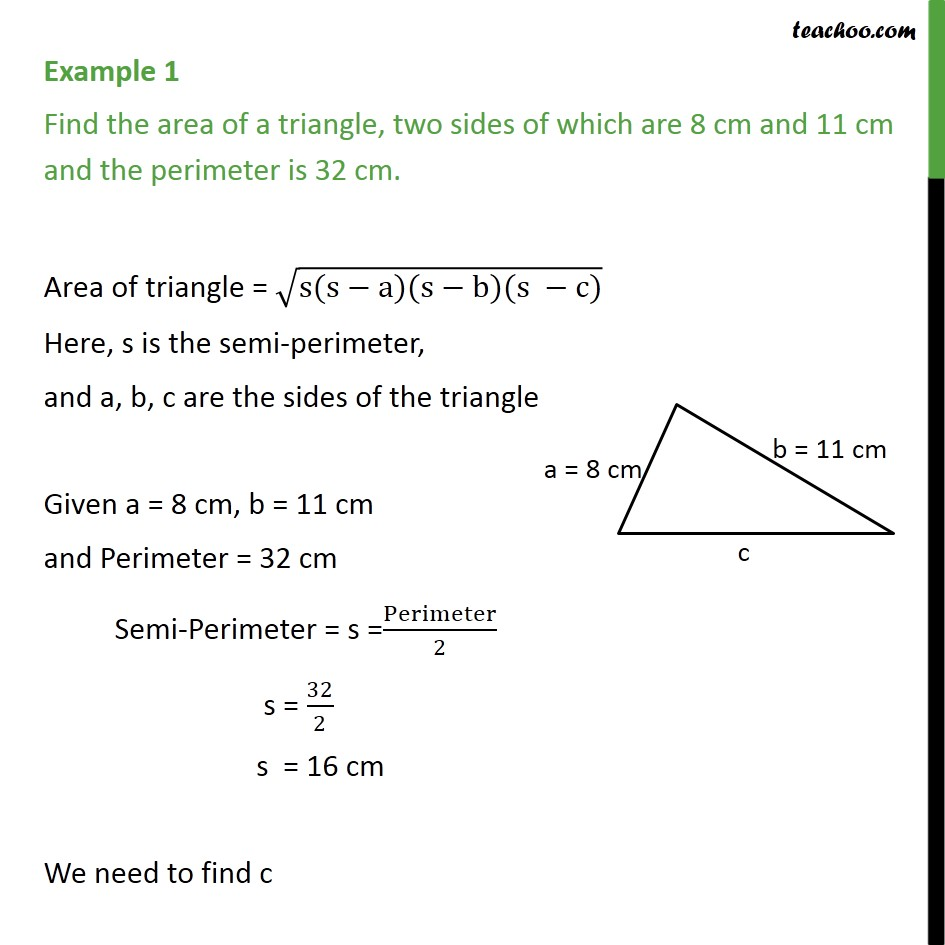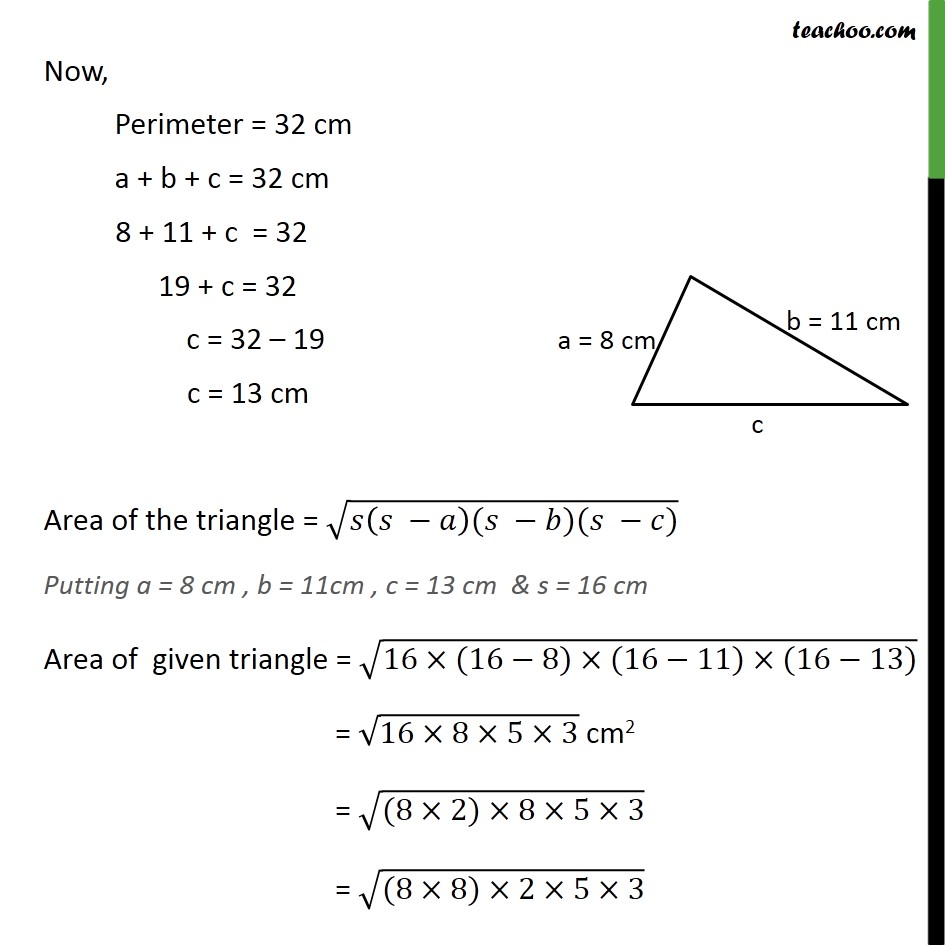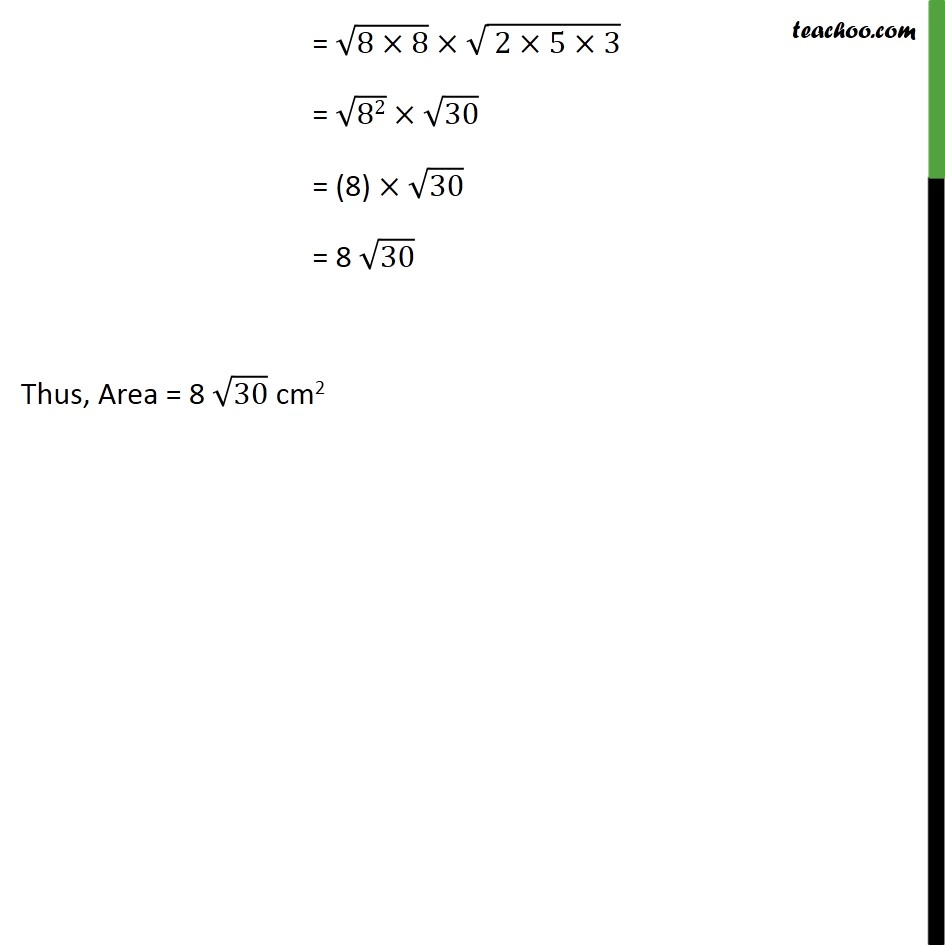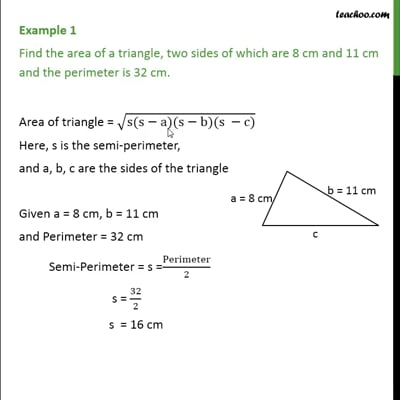Examples

Chapter 12 Class 9 Herons Formula
Serial order wiseThis video is only available for Teachoo black users

Maths Crash Course - Live lectures + all videos + Real time Doubt solving!

### Transcript

Example 1 Find the area of a triangle, two sides of which are 8 cm and 11 cm and the perimeter is 32 cm. Area of triangle = √(s(s−a)(s−b)(s −c)) Here, s is the semi-perimeter, and a, b, c are the sides of the triangle Given a = 8 cm, b = 11 cm and Perimeter = 32 cm Semi-Perimeter = s =Perimeter/2 s = 32/2 s = 16 cm We need to find c Now, Perimeter = 32 cm a + b + c = 32 cm 8 + 11 + c = 32 19 + c = 32 c = 32 – 19 c = 13 cm Area of the triangle = √(𝑠(𝑠 −𝑎)(𝑠 −𝑏)(𝑠 −𝑐)) Putting a = 8 cm , b = 11cm , c = 13 cm & s = 16 cm Area of given triangle = √(16×(16−8)×(16−11)×(16−13)) = √(16×8×5×3) cm2 = √((8×2)×8×5×3) = √((8×8)×2×5×3) = √(8×8)×√( 2×5×3) = √82×√30 = (8) ×√30 = 8 √30 Thus, Area = 8 √30 cm2